# Maths - Matrix to Axis-Angle - Sample Orientations

## Sample Rotations

In order to try to explain things and give some examples we can try I thought it might help to show the rotations for a finite subset of the rotation group. We will use the set of rotations of a cube onto itself, this is a permutation group which gives 24 possible rotations as explaned on this page.

In the following table we will need to know what quadrant the results are in, so I have taken some sample results from Math.atan2

heading applied first giving 4 possible orientations:reference orientation

 1 0 0 0 1 0 0 0 1

angle = 2 * acos(sqrt(1.0 + m00 + m11 + m22) / 2.0)
x = m21 - m12
y = m02 - m20
z = m10 - m01

angle = 2 * acos(sqrt(4) / 2.0) =0
x = 0
y = 0
z = 0

angle = 0 degrees
axis = 1,0,0rotate by 90 degrees about y axis

 0 0 1 0 1 0 -1 0 0

angle = 2 * acos(sqrt(1.0 + m00 + m11 + m22) / 2.0)
x = m21 - m12
y = m02 - m20
z = m10 - m01

angle = 2 * acos(sqrt(2) / 2.0) = 90deg
x = 0
y = 2
z = 0

angle = 90 degrees
axis = 0,1,0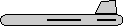rotate by 180 degrees about y axis

 -1 0 0 0 1 0 0 0 -1

angle = 2 * acos(sqrt(1.0 + m00 + m11 + m22) / 2.0)
x = m21 - m12
y = m02 - m20
z = m10 - m01

angle = 2 * acos(sqrt(1.0 + m00 + m11 + m22) / 2.0)
x = m21 - m12
y = m02 - m20
z = m10 - m01

angle = 180 degrees
axis = 0,1,0rotate by 270 degrees about y axis

 0 0 -1 0 1 0 1 0 0

angle = 2 * acos(sqrt(1.0 + m00 + m11 + m22) / 2.0)
x = m21 - m12
y = m02 - m20
z = m10 - m01

angle = 2 * acos(sqrt(1.0 + m00 + m11 + m22) / 2.0)
x = m21 - m12
y = m02 - m20
z = m10 - m01

angle = 90 degrees
axis = 0,-1,0

or

angle = -90 degrees
axis = 0,1,0

Then apply attitude +90 degrees for each of the above: (note: that if we went on to apply bank to these it would just rotate between these values, the straight up and streight down orientations are known as singularities because they can be fully defined without using the bank value)0 -1 0 1 0 0 0 0 1

angle = 2 * acos(sqrt(1.0 + m00 + m11 + m22) / 2.0)
x = m21 - m12
y = m02 - m20
z = m10 - m01

angle = 2 * acos(sqrt(1.0 + m00 + m11 + m22) / 2.0)
x = m21 - m12
y = m02 - m20
z = m10 - m01

angle = 90 degrees
axis = 0,0,10 0 1 1 0 0 0 1 0

angle = 2 * acos(sqrt(1.0 + m00 + m11 + m22) / 2.0)
x = m21 - m12
y = m02 - m20
z = m10 - m01

angle = 2 * acos(sqrt(1.0) / 2.0) =2*60deg = 120 deg
x = 1
y = 1
z = 1

angle = 120 degrees
axis = 0.5774,0.5774,0.57740 1 0 1 0 0 0 0 -1

angle = 2 * acos(sqrt(1.0 + m00 + m11 + m22) / 2.0)
x = m21 - m12
y = m02 - m20
z = m10 - m01

angle = 2 * acos(sqrt(1.0 + m00 + m11 + m22) / 2.0)
x = m21 - m12
y = m02 - m20
z = m10 - m01

angle = 180 degrees
axis = 0.7071,0.7071,00 0 -1 1 0 0 0 -1 0

angle = 2 * acos(sqrt(1.0 + m00 + m11 + m22) / 2.0)
x = m21 - m12
y = m02 - m20
z = m10 - m01

angle = 2 * acos(sqrt(1.0 + m00 + m11 + m22) / 2.0)
x = m21 - m12
y = m02 - m20
z = m10 - m01

angle = 120 degrees
axis = -0.5774,-0.5774,0.5774

Or instead apply attitude -90 degrees (also a singularity):0 1 0 -1 0 0 0 0 1

angle = 2 * acos(sqrt(1.0 + m00 + m11 + m22) / 2.0)
x = m21 - m12
y = m02 - m20
z = m10 - m01

angle = 2 * acos(sqrt(1.0 + m00 + m11 + m22) / 2.0)
x = m21 - m12
y = m02 - m20
z = m10 - m01

angle = 90 degrees
axis = 0,0,-1

(equivilant rotation to:
angle = -90 degrees
axis = 0,0,1)0 0 1 -1 0 0 0 -1 0

angle = 2 * acos(sqrt(1.0 + m00 + m11 + m22) / 2.0)
x = m21 - m12
y = m02 - m20
z = m10 - m01

angle = 2 * acos(sqrt(1.0 + m00 + m11 + m22) / 2.0)
x = m21 - m12
y = m02 - m20
z = m10 - m01

angle = 120 degrees
axis = -0.5774,0.5774,-0.5774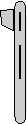0 -1 0 -1 0 0 0 0 -1

angle = 2 * acos(sqrt(1.0 + m00 + m11 + m22) / 2.0)
x = m21 - m12
y = m02 - m20
z = m10 - m01

angle = 2 * acos(sqrt(1.0 + m00 + m11 + m22) / 2.0)
x = m21 - m12
y = m02 - m20
z = m10 - m01

angle = 180 degrees
axis = -0.7071,0.7071,0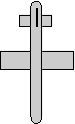0 0 -1 -1 0 0 0 1 0

angle = 2 * acos(sqrt(1.0 + m00 + m11 + m22) / 2.0)
x = m21 - m12
y = m02 - m20
z = m10 - m01

angle = 2 * acos(sqrt(1.0 + m00 + m11 + m22) / 2.0)
x = m21 - m12
y = m02 - m20
z = m10 - m01

angle = 120 degrees
axis = 0.5774,-0.5774,-0.5774

Normally we dont go beond attitude + or - 90 degrees because thes are singularities, instead apply bank +90 degrees:1 0 0 0 0 -1 0 1 0

angle = 2 * acos(sqrt(1.0 + m00 + m11 + m22) / 2.0)
x = m21 - m12
y = m02 - m20
z = m10 - m01

angle = 2 * acos(sqrt(1.0 + m00 + m11 + m22) / 2.0)
x = m21 - m12
y = m02 - m20
z = m10 - m01

angle = 90 degrees
axis = 1,0,00 1 0 0 0 -1 -1 0 0

angle = 2 * acos(sqrt(1.0 + m00 + m11 + m22) / 2.0)
x = m21 - m12
y = m02 - m20
z = m10 - m01

angle = 2 * acos(sqrt(1.0 + m00 + m11 + m22) / 2.0)
x = m21 - m12
y = m02 - m20
z = m10 - m01

angle = 120 degrees
axis = 0.5774,0.5774,-0.5774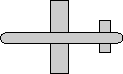-1 0 0 0 0 -1 0 -1 0

angle = 2 * acos(sqrt(1.0 + m00 + m11 + m22) / 2.0)
x = m21 - m12
y = m02 - m20
z = m10 - m01

angle = 2 * acos(sqrt(1.0 + m00 + m11 + m22) / 2.0)
x = m21 - m12
y = m02 - m20
z = m10 - m01

angle = 180 degrees
axis = 0,0.7071,-0.70710 -1 0 0 0 -1 1 0 0

angle = 2 * acos(sqrt(1.0 + m00 + m11 + m22) / 2.0)
x = m21 - m12
y = m02 - m20
z = m10 - m01

angle = 2 * acos(sqrt(1.0 + m00 + m11 + m22) / 2.0)
x = m21 - m12
y = m02 - m20
z = m10 - m01

angle = 120 degrees
axis = 0.5774,-0.5774,0.5774

Apply bank +180 degrees:1 0 0 0 -1 0 0 0 -1

angle = 2 * acos(sqrt(1.0 + m00 + m11 + m22) / 2.0)
x = m21 - m12
y = m02 - m20
z = m10 - m01

angle = 2 * acos(sqrt(1.0 + m00 + m11 + m22) / 2.0)
x = m21 - m12
y = m02 - m20
z = m10 - m01

angle = 180 degrees
axis = 1,0,00 0 -1 0 -1 0 -1 0 0

angle = 2 * acos(sqrt(1.0 + m00 + m11 + m22) / 2.0)
x = m21 - m12
y = m02 - m20
z = m10 - m01

angle = 2 * acos(sqrt(1.0 + m00 + m11 + m22) / 2.0)
x = m21 - m12
y = m02 - m20
z = m10 - m01

angle = 180 degrees
axis = 0.7071,0,-0.7071-1 0 0 0 -1 0 0 0 1

angle = 2 * acos(sqrt(1.0 + m00 + m11 + m22) / 2.0)
x = m21 - m12
y = m02 - m20
z = m10 - m01

angle = 2 * acos(sqrt(1.0 + m00 + m11 + m22) / 2.0)
x = m21 - m12
y = m02 - m20
z = m10 - m01

angle = 180 degrees
axis = 0,0,10 0 1 0 -1 0 1 0 0

angle = 2 * acos(sqrt(1.0 + m00 + m11 + m22) / 2.0)
x = m21 - m12
y = m02 - m20
z = m10 - m01

angle = 2 * acos(sqrt(1.0 + m00 + m11 + m22) / 2.0)
x = m21 - m12
y = m02 - m20
z = m10 - m01

angle = 180 degrees
axis = 0.7071,0,0.7071

Apply bank -90 degrees:1 0 0 0 0 1 0 -1 0

angle = 2 * acos(sqrt(1.0 + m00 + m11 + m22) / 2.0)
x = m21 - m12
y = m02 - m20
z = m10 - m01

angle = 2 * acos(sqrt(1.0 + m00 + m11 + m22) / 2.0)
x = m21 - m12
y = m02 - m20
z = m10 - m01

angle = 90 degrees
axis = -1,0,0

(equivilant rotation to:
angle = -90 degrees
axis = 1,0,0)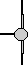0 -1 0 0 0 1 -1 0 0

angle = 2 * acos(sqrt(1.0 + m00 + m11 + m22) / 2.0)
x = m21 - m12
y = m02 - m20
z = m10 - m01

angle = 2 * acos(sqrt(1.0 + m00 + m11 + m22) / 2.0)
x = m21 - m12
y = m02 - m20
z = m10 - m01

angle = 120 degrees
axis = -0.5774,0.5774,0.5774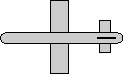-1 0 0 0 0 1 0 1 0

angle = 2 * acos(sqrt(1.0 + m00 + m11 + m22) / 2.0)
x = m21 - m12
y = m02 - m20
z = m10 - m01

angle = 2 * acos(sqrt(1.0 + m00 + m11 + m22) / 2.0)
x = m21 - m12
y = m02 - m20
z = m10 - m01

angle = 180 degrees
axis = 0,0.7071,0.70710 1 0 0 0 1 1 0 0

angle = 2 * acos(sqrt(1.0 + m00 + m11 + m22) / 2.0)
x = m21 - m12
y = m02 - m20
z = m10 - m01

angle = 2 * acos(sqrt(1.0 + m00 + m11 + m22) / 2.0)
x = m21 - m12
y = m02 - m20
z = m10 - m01

angle = 120 degrees
axis = -0.5774,-0.5774,-0.5774